0
98

Download CMAT Matrix Arrangement questions with solutions PDF by Cracku. Practice CMAT solved Matrix Arrangement Questions paper tests, which are the practice question to have a firm grasp on the Matrix Arrangement topic in the CMAT exam. Top 20 very Important Matrix Arrangement Questions for CMAT based on the questions asked in previous exam papers. Click on the link below to download the Matrix Arrangement Questions for CMAT PDF with detailed solutions.

Question 1: A word is represented by only one set of numbers as given in any one of the alternatives. The sets of numbers given in the alternatives are represented by two classes of alphabets as in two matrices given below. The columns and rows of matrix I and II are numbered from 0 to 4.A letter from these matrices can be represented first by its row and next by its column,e.g., ‘A’ can be represented by 24, 31 etc. and ‘P’ can be represented by 11, 32, etc. Identify the set for the letters AELO.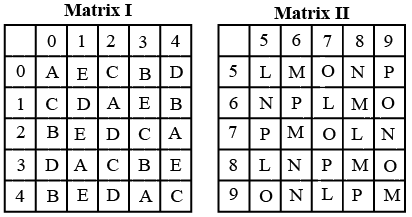a) 31, 00, 23, 22

b) 43, 01, 12, 42

c) 12, 34, 30, 02

d) 12, 30, 42, 14

Solution:

Trying the sequences in different options helps us arrive at the right combination of numbers for the word AELO. It is option C.

Question 2: A word is represented by only one set of numbers as given in any one of the alternatives. The sets of numbers given in the alternatives are represented by two classes of alphabets as in the two matrices given below. The columns and rows of Matrix I are numbered from 0 to 4 and that of Matrix II are numbered from 5 to 9. A letter from these matrices can be represented first by its row and next by its column, eg. ‘A’ can be represented by 13, 76, etc., and ‘G’ can be represented by 22, 65, etc. Similarly, you have ta identify the set for the word ‘PUBLIC’.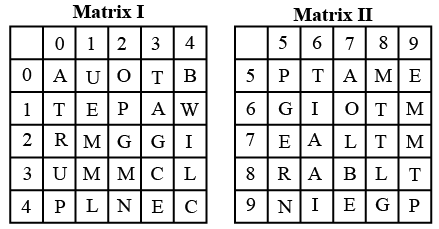a) 12, 30, 87, 41, 66, 33

b) 99, 30, 87, 77, 23, 44

c) 55, 01, 87, 98, 34, 87

d) 40, 30, 87, 89, 24, 43

Solution:

From the given matrices,

P can be represented as 12, 40, 55, 99

U can be represented as 01, 30

B can be represented as 04, 87

L can be represented as 34, 41, 88

I can be represented as 24, 66, 91

C can be represented as 33, 44

From the given options, PUBLIC can be represented as 12, 30, 87, 41, 66, 33

Hence, the correct answer is Option A

Question 3: A word is represented by only one set of numbers as given in any one of the alternatives. The sets of numbers given in the alternatives are represented by two classes of alphabets as in two matrices given below. The columns and rows of Matrix I are numbered from 0 to 4 and that of Matrix II are numbered from 5 to 9. A letter from these matrices can be represented first by its row and next by its column, e.g. ‘M’ can be represented by 01, 14 etc., and ‘S’ can be represented by 58, 77 etc. Similaraly, you have to identify the set the word ‘ROHAN’.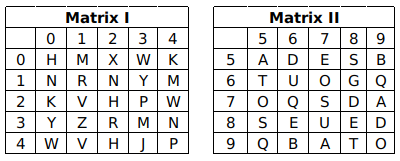a) 11, 57, 00, 55, 12

b) 11, 75, 00, 55, 10

c) 32, 75, 21, 55, 10

d) 32, 67, 41, 55, 12

Solution:

R = 11, 32,
O = 75, 67, 99
H = 00, 22,
A = 55, 97, 79
N = 10, 12, 34,

From option B,
ROHAN = 11, 75, 00, 55, 10

$\therefore$ The correct answer is option B.

Question 4: A word is represented by only one set of numbers as given in any one of the alternatives. The sets of numbers given in the alternatives are represented by two classes of alphabets as shown in the given two matrices. The columns and rows of Matrix-I are numbered from 0 to 4 and that of Matrix-It are numbered from 5 to 9. A letter from these matrices can be represented first by Rs row and next by Its column, for example ‘N’ can be represented by 00,12 etc and ‘C’ can be represented by 95, 76 etc. Similarly, you have to identify the set for the word ‘TYRES’.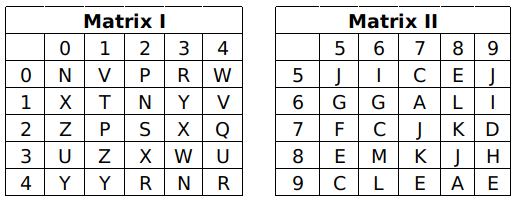a) 21,40,04,69,01

b) 12,13,41,55,69

c) 11,41,44,85,22

d) 31,01,65,58,41

Solution:

T = 11,

Y =40, 41,

R=44, 42

E=85, 58

S=22

TYRES = 11, 41, 44, 85, 22

$\therefore$ The correct answer is option C.

Question 5: A word is represented by only one set of numbers as given in any one of the alternatives. The sets of numbers given in the alternatives are represented by two classes of alphabets as shown in the given two matrices. The columns and rows of Matrix-I are numbered from 0 to 4 and that of Matrix-Il are numbered from 5 to 9. A Setter from these matrices can be represented first by its row and next by its column, for example ‘X’ can be represented by 30, 41 etc and ‘G’ can be represented by SS. 87 etc. Similarly, you have to identify the set for the word ‘RUGBY.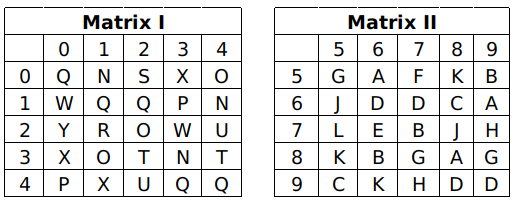a) 01, 34, 22, 10, 75

b) 40, 31, 41, 68, 01

c) 21, 24, 89, 77, 20

d) 23, 95, 14, 20, 79

Solution:

R = 21

U = 42, 24

G = 55, 89

B = 77

Y = 20

RUGBY = 21, 24, 89, 77, 20

$\therefore$ The correct answer is option C.

Question 6: A word is represented by only one set of numbers as given in any one of the alternatives. The sets of numbers given in the alternatives are represented by two classes of alphabets as shown in the given two matrices. The columns and rows of Matrix-I are numbered from 0 to 4 and that of Matrix-II are numbered from 5 to 9. A letter from these matrices can be represented first by its row and next by its column, for example ‘T’ can be represented by 10, 32 etc and ‘A’ can be represented by 65, 56 etc. Similarly, you have to identify the set for the word ‘WOVEN’.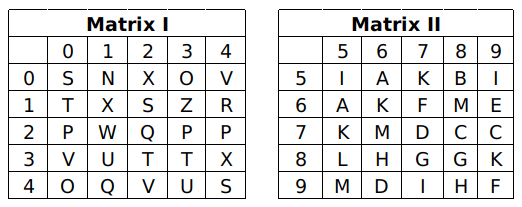a) 12,13,41,55,69

b) 31,01,65,58,41

c) 24,20,40,31,76

d) 21,40,04,69,01

Solution:

W = 21

O = 03, 40

V = 30, 04

E = 69

N = 01

WOVEN = 21, 40, 04, 69, 01

$\therefore$ The correct answer is option D.

Question 7: A word is represented by only one set of numbers as given in any one of the alternatives. The sets of numbers given in the alternatives are represented by two classes of alphabets as shown in the given two matrices, The columns and rows of Matrix-I are numbered from 0 to 4 and that of Matrix-lI are numbered from 5 to 9. letter from these matrices can be represented first by its row and next by its column, for example “U’ can be represented by 30, 41 etc and ‘E’ can be represented by 85, 67 etc. Similarly, you have to identify the set for the word SWORD”.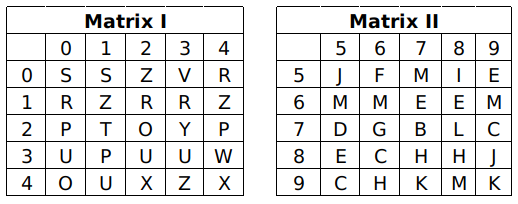a) 40,31,41,68,01

b) 23,95,14,20,79

c) 11,41,44,85,22

d) 01,34,22,10,75

Solution:

S = 00, 01,
W = 34
O = 40, 22
R = 10, 12, 13
D = 75

By the option D,
‘SWORD’ represented by 01, 34, 22, 10, 75
$\therefore$ The correct answer is option D.

Question 8: A word is represented by only one set of numbers as given in any one of the alternatives. The sets of numbers given in the alternatives are represented by two classes of alphabets as shown in the given two matrices. The columns and rows of Matrix-I are numbered from 0 to 4 and that of Matrix-II are numbered from 5 to 9. A letter from these matrices can be represented first by its row and next by its column, for example ‘A’ can be represented by 34, 12 etc and ‘X’ can be represented by 89, 97 etc. Similarly, you have to identify the set for the word ‘WHEY’.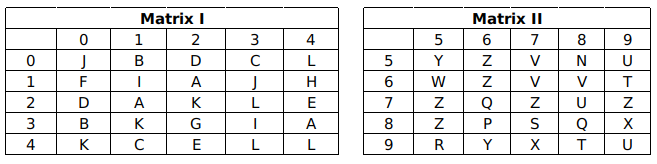a) 56, 69, 42, 78

b) 99, 24, 01, 67

c) 65, 14, 42, 96

d) 55, 89, 04, 23

Solution:

From the given matrices,

W can be represented as 65

H can be represented as 14

E can be represented as 24,42

Y can be represented as 55

From the given options, WHEY can be represented as 65, 14, 42, 96

Hence, the correct answer is Option C

Question 9: A word is represented by only one set of numbers as given in any one of the alternatives. The sets of numbers given in the alternatives are represented by two classes of alphabets as shown in the given two matrices. The columns and rows of Matrix-I are numbered from 0 to 4 and that of Matrix-II are numbered from 5 to 9. A letter from these matrices can be represented first by its row and next by its column, for example ‘G’ can be represented by 31, 13 etc and ‘X’ can be represented by 89, 75 etc. Similarly, you have to identify the set for the word ‘AWRY’.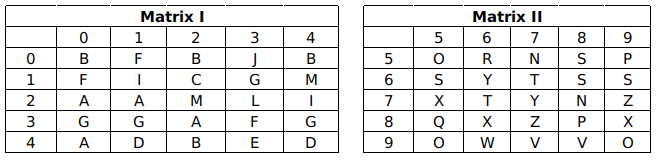a) 21,78,42,56

b) 32,54,98,31

c) 33,77,21,12

d) 20,96,56,77

Solution:

From the given matrices,

A can be represented as 20, 21, 32, 40

W can be represented as 96

R can be represented as 56

Y can be represented as 66, 77

From the given options, AWRY can be represented as 20,96,56,77

Hence, the correct answer is Option D

Question 10: A word is represented by only one set of numbers as given in any one of the alternatives. The sets of numbers given in the alternatives are represented by two classes of alphabets as shown in the given two matrices. The columns and rows of Matrix-I are numbered from 0 to 4 and that of Matrix-II are numbered from 5 to 9. A letter from these matrices can be represented first by its row and next by its column, for example ‘J’ can be represented by 34, 20 etc and ‘Q’ can be represented by 78, 97 etc. Similarly, you have to identify the set for the word ‘INKY’.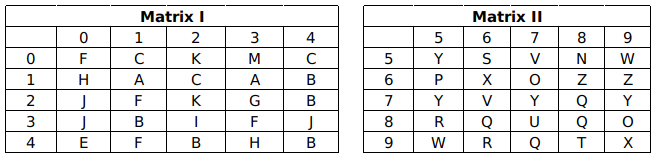a) 32,58,02,75

b) 34,85,98,10

c) 43,89,04,55

d) 11,67,03,65

Solution:

From the given matrices,

I can be represented as 32

N can be represented as 58

K can be represented as 02, 22

Y can be represented as 55, 75

From the given options, INKY can be represented as 32,58,02,75

Hence, the correct answer is Option A

Question 11: A word is represented by only one set of numbers as given in any one of the alternatives. The sets of numbers given in the alternatives are represented by two classes of alphabets as shown in the given two matrices. The columns and rows of Matrix-I are numbered from 0 to 4 and that of Matrix-II are numbered from 5 to 9. A letter from these matrices can be represented first by its row and next by its column, for example ‘B’ can be represented by 43, 31 etc and ‘X’ can be represented by 76, 96 etc. Similarly, you have to identify the set for the word ‘KEPT’.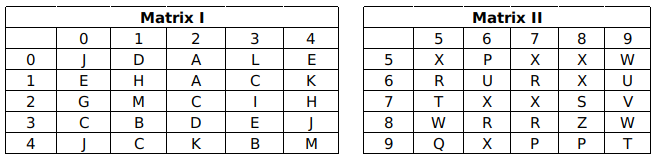a) 01,56,89,43

b) 41,01,78,88

c) 42,58,02,56

d) 14,10,56,99

Solution:

From the given matrices,

K can be represented as 14, 42

E can be represented as 04, 10, 33

P can be represented as 56, 97, 98

T can be represented as 75, 99

From the given options, KEPT can be represented as 14,10,56,99

Hence, the correct answer is Option D

Question 12: A word is represented by only one set of numbers as given in any one of the alternatives. The sets of numbers given in the alternatives are represented by two classes of alphabets as shown in the given two matrices. The columns and rows of Matrix-I are numbered from 0 to 4 and that of Matrix-II are numbered from S to 9. A letter from these matrices can be represented first by its row and next by its column, for example ‘K’ can be represented by 32, 01 etc and ‘R’ can be represented by 79, 99 etc. Similarly, you have to identify the set for the word ‘GLUE’.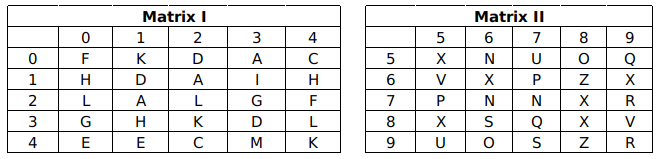a) 30, 33, 78, 43

b) 21, 67, 98, 32

c) 23, 34, 57, 40

d) 33, 87, 12, 67

Solution:

From the given matrices,

G can be represented as 23, 30

L can be represented as 20, 22, 34

U can be represented as 57, 95

E can be represented as 40, 41

From the given options, GLUE can be represented as 23, 34, 57, 40

Hence, the correct answer is Option C

Question 13: A word is represented by only one set of numbers as given in any one of the alternatives. The sets of numbers given in the alternatives are  represented by two classes of alphabets as shown in the given two matrices. The columns and rows of Matrix-I are numbered from 0 to 4 and that of Matrix-II are numbered from 5 to 9. A letter from these matrices can be represented first by its row and next by its column, for example ‘H’ can be represented by 21, 43 etc and ‘R’ can be represented by 96, 87 etc. Similarly, you have to identify the set for the word ‘POEM’.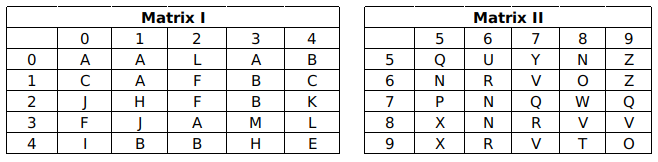a) 76,69,01,33

b) 56,88,23,41

c) 89,56,44,02

d) 75,68,44,33

Solution:

From the given matrices,

P can be represented as 75

O can be represented as 68

E can be represented as 44

M can be represented as 33

From the given options, POEM can be represented as 75,68,44,33

Hence, the correct answer is Option D

Question 14: A word is represented by only one set of numbers as given in any one of the alternatives. The sets of numbers given in the alternatives are represented by two classes of alphabets as shown in the given two matrices. The columns and rows of Matrix-I are numbered from 0 to 4 and that of Matrix-II are numbered from 5 to 9. A letter from these matrices can be represented first by its row and next by its column, for example ‘E’ can be represented by 32, 12 etc and ‘X’ can be represented by 87, 79 etc. Similarly, you have to identify the set for the word ‘MYTH’.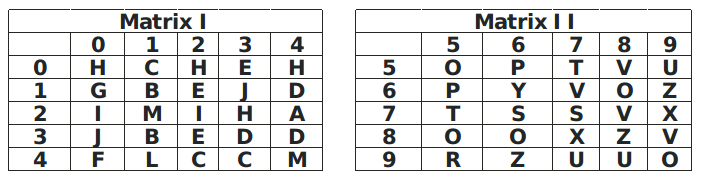a) 44,89,57,23

b) 20,89,66,43

c) 21,66,75,04

d) 32,75,43,66

Solution:

From the given matrices,

M can be represented as 21, 44

Y can be represented as 66

T can be represented as 57, 75

H can be represented as 00, 02, 04, 23

From the given options, MYTH can be represented as 21,66,75,04

Hence, the correct answer is Option C

Question 15: A word is represented by only one set of numbers as given in any one of the alternatives. The sets of numbers given in the alternatives are represented by two classes of alphabets as shown in the given two matrices, The columns and rows of Matrix-I are numbered from 0 to 4 and that of Matrix-II are numbered from 5 to 9. A letter from these matrices can be represented first by its row and next by its column, for example ‘P’ can be represented by 79, 59 etc and ‘I’ can be represented by 31, 20 etc. Similarly, you have to identify the set for the word ‘TURF’.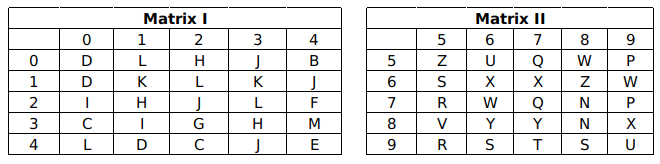a) 67, 99, 75, 23

b) 89, 56, 41, 34

c) 87, 97, 42, 12

d) 97, 56, 75, 24

Solution:

From the given matrices,

T can be represented as 97

U can be represented as 56, 99

R can be represented as 75, 95

F can be represented as 24

From the given options, TURF can be represented as 97, 56, 75, 24

Hence, the correct answer is Option D

Question 16: A word is represented by only one set of numbers as given in any one of the alternatives. The sets of numbers given in the alternatives are represented by two classes of alphabets as shown in the given two matrices. The columns and rows of Matrix-I are numbered from 0 to 4 and that of Matrix-II are numbered from 5 to 9. A letter from these matrices can be represented first by its row and next by its column, for example ‘C’ can be represented by 43, 41 etc and ‘O’ can be represented by 97, 78 etc. Similarly, you have to identify the set for the word ‘SPAN’.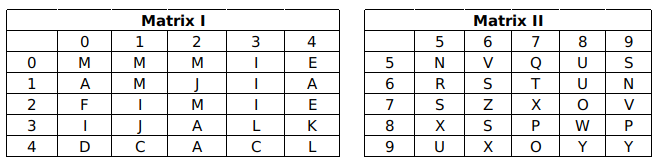a) 66,87,33,56

b) 59,78,42,31

c) 86,89,32,55

d) 78,43,22,98

Solution:

From the given matrices,

S can be represented as 59, 66, 75, 86

P can be represented as 87, 89

A can be represented as 10, 14, 32, 42

N can be represented as 55, 69

From the given options, SPAN can be represented as 86,89,32,55

Hence, the correct answer is Option C

Question 17: A word is represented by only one set of numbers as given in any one of the alternatives. The sets of numbers given in the alternatives are represented by two classes of alphabets as in two matrices given below. The columns and rows of Matrix-I are numbered from 0 to 4 and that of Matrix-2 are numbered from 5 to 9. A letter from these matrices can be represented first by its row and next by its column, e.g., ‘M’ can be represented by 01, 10 etc., and ‘R’ can be represented by 58,85 etc. Similarly, you have to identify the set for the word ‘NOW’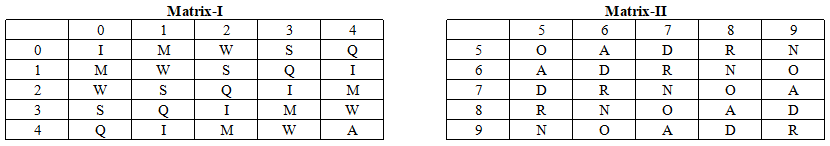a) 86, 58, 11

b) 55, 78, 11

c) 95, 55, 34

d) 95, 67, 02

Solution:

From the given matrices,

N can be represented as 59, 68, 77, 86, 95

O can be represented as 55, 69, 78, 87, 96

W can be represented as 02, 11, 20, 34, 43

From the given options, NOW can be represented as 95, 55, 34

Hence, the correct answer is Option C

Question 18: A word is represented by only one set of numbers as given in any one of the alternatives. The sets of numbers given in the alternatives are represented by two classes of alphabets as in two matrices given below. The columns and rows of Matrix I are numbered from 0 to 4 and that of Matrix II are numbered from 5 to 9. A letter from these matrices can be represented first by its row and next by its column, e.g., M can be represented by 01, 10 etc., and A can be represented by 56, 65 etc. Similarly, you have to identify the set for the word ROD.a) 67, 96, 57

b) 56, 66, 86

c) 58, 66, 78

d) 58, 69, 65

Solution:

From the given matrices,

R can be represented as 58, 67, 76, 85, 99

O can be represented as 55, 69, 78, 87, 96

D can be represented as 57, 66, 75, 89, 98

From the given options, ROD can be represented as 67, 96, 57

Hence, the correct answer is Option A

Question 19: A word is represented by only one set of numbers as given in any one of the alternatives. The sets of numbers given in the alternatives are represented by two classes of alphabet as shown in the given two matrices. The columns and rows of Matrix-I are numbered from 0 to 4 and that of Matrix-II are numbered from 5 to 9. A letter from these matrices can be represented first by its row and next by its column., for example, ‘R’ can be represented by 10, 42, etc., and “I’ can be represented by 75, 99, etc. Similarly, you have to identify the set for the word “BOND”.

a) 00, 97, 56, 59

b) 13, 65, 66, 22

c) 24, 78, 33, 13

d) 32, 89, 59, 20

Solution:

(A) : 00, 97, 56, 59 = BOON

(B) : 13, 65, 66, 22 = BONE

(C) : 24, 78, 33, 13 = BODB

(D) : 32, 89, 59, 20 = BOND

=> Ans – (D)

Question 20: A word is represented by only one set of numbers as given in any one of the alternatives. The sets of numbers given in the alternatives are represented by two classes of alphabet as shown in the given two matrices. The columns and rows of Matrix-I are numbered from 0 to 4 and that of Matrix-II are numbered from 5 to 9. A letter from these matrices can be represented first by its row and next by its column., for example, ‘B’ can be represented by 04, 20, etc., and “I’ can be represented by 56, 95, etc. Similarly, you have to identify the set for the word “SLAP”.

a) 57, 00, 20, 13

b) 65, 21, 75, 66

c) 78, 34, 43, 86

d) 96, 42, 98, 77